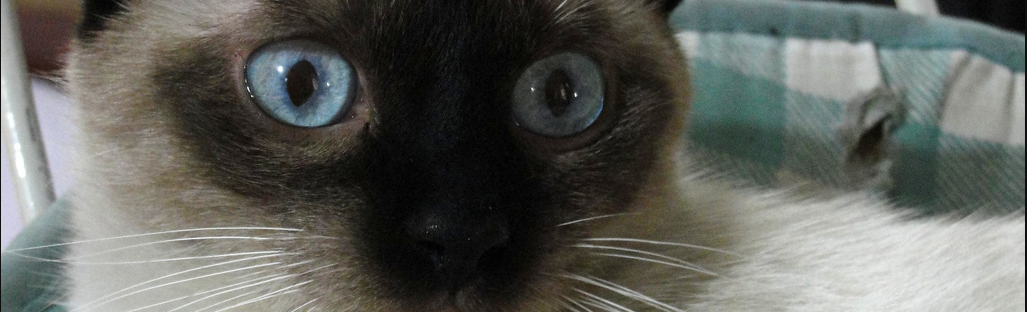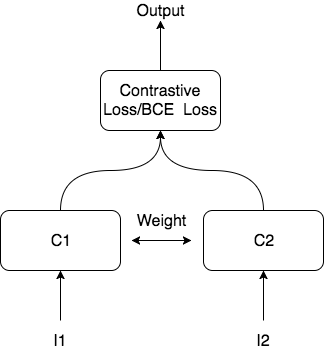# LFW face classification using Siamese Network

Nov 2017 Linhao Jin

Labeled Faces in the Wild is a dataset for facial recognition that contains more than 13,000 images of faces collected from the web. Here we use Siamese Network in Pytorch with two loss functions - Contrasive Loss and BCE Loss to do the classify if a pair of images of faces is the same person.Consider a Convolutional Neural Network (CNN), denoted by C, that takes as input a single image I and outputs a feature vector fN, where f is simply the output of the final fully connected layer that contains N nodes (and hence, N numbers are produced). In other words, C(I)=fN. A Siamese Network is a CNN that takes two separate image inputs, I1 and I2, and both images go through the same exact CNN C (e.g., this is what’s called “shared weights”), and so we can say: C(I1) = f1 and C(I2) = f2. We can process the images in one pass, and then we can “play games” with the features that are output to learn what it means for two images (of faces) represented by their respective 'latent embeddings' (f) to be the same person/identity or not.

The network here contains 19 layers. To train the network on GPU, it requires at least 12G of GPU memory. I only use 4000 labeled images in this project.

The results shows that BCE model overfits dataset (99% accuracy), but the Contrastive accuracy can go up to 80%. I only train 20 epochs, it should go higher if I increase the epochs.

Codes available at: https://github.com/Jin-Linhao/Siamese_lfw_pytorch